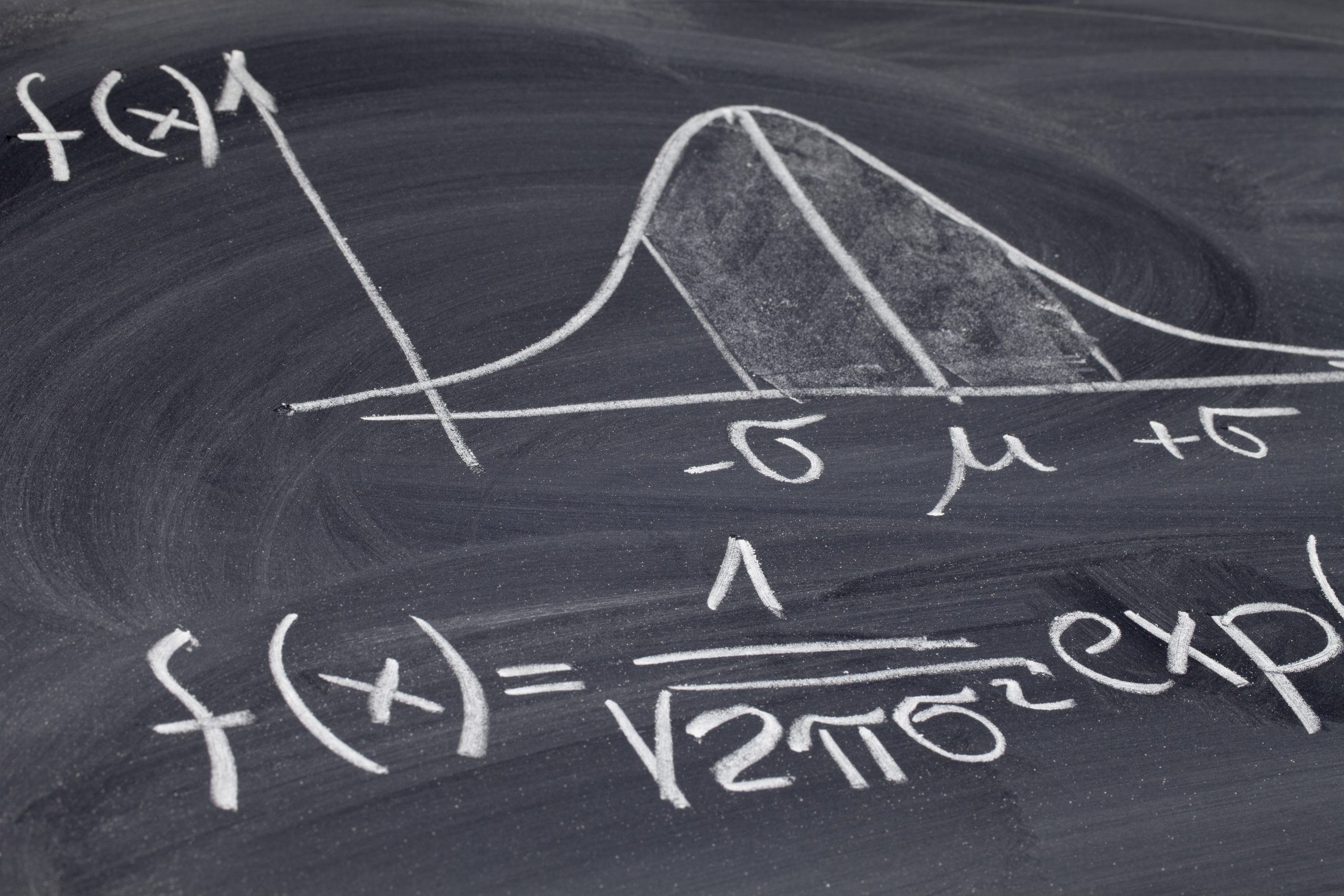# Statistics Intro: Financial Maths Part II

A strong understanding of statistics shouldn't just be the reserve of analysts. Statistical concepts are crucial to anyone working in finance. Dive into this pathway to learn how to read market trends and make better informed investment decisions.

Pathway

Technical FoundationsAccess this and 100s of other videos by signing up for a 14-day free trial.

#### CPD credits earned

1.5#### Certificates

Watch all the videos and pass the test to obtain a certificate showing your completion of this Pathway. Certificates can be shared directly to your LinkedIn profile and social media accounts.

9 videos • 42 minutes

•Financial maths### Measures of Central Tendency

Abdulla outlines measures of Central Tendency, and explains the differences between calculating the Mean, Median and Mode.

Abdulla Javeri03:18

•Financial maths### The Arithmetic Mean

Following an overview of the mean, the median and the mode. Abdulla focuses on the arithmetic mean to determine its value as  an appropriate measure.

Abdulla Javeri04:16

•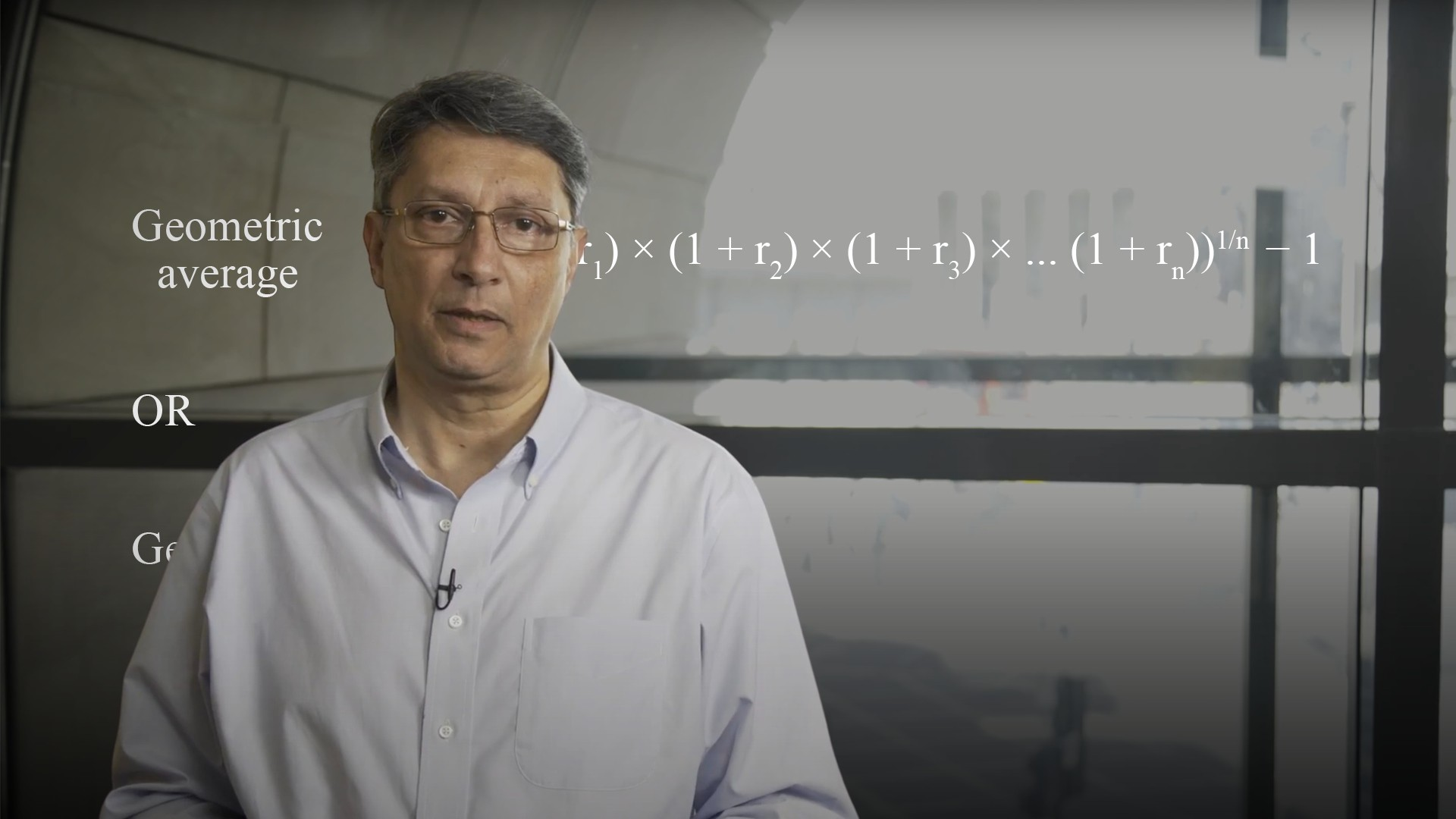Financial maths### The Geometric Mean

Abdulla outlines how to use and calculate the geometric average or geometric mean, also referred to as CAGR- the compounded average growth rate.

Abdulla Javeri04:41

•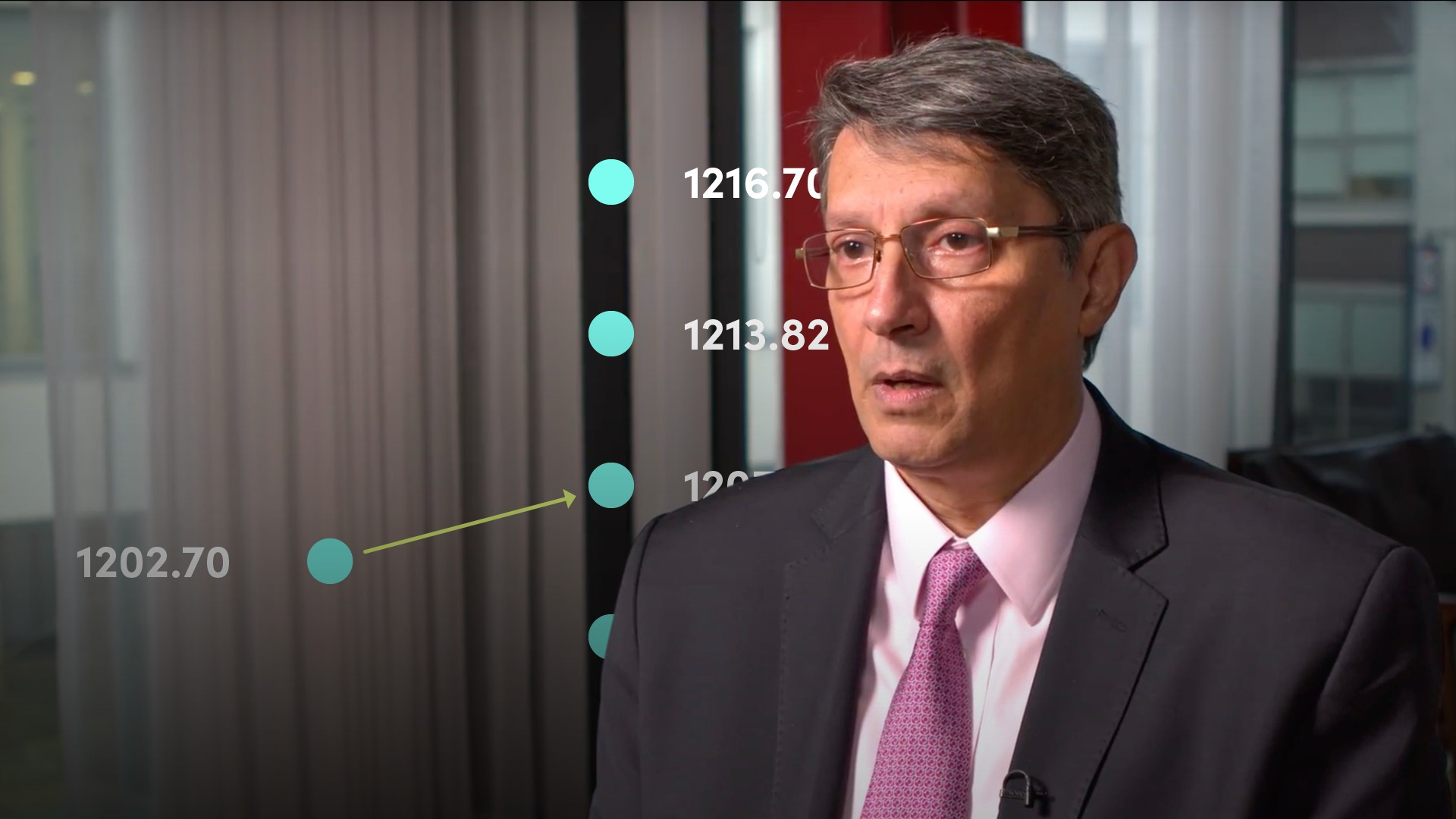Financial maths### Measures of Dispersion

In this video, Abdulla covers measures of dispersion to determine how the data is spread around the central point.

Abdulla Javeri06:35

•Financial maths### Calculating Discrete Holding Period Returns

As investors or traders, the main objective is to make a profit. Returns can be calculated for a holding period or on an annualised basis, and on a continuously compounded basis or a discrete basis. In this video, Abdulla will provide a formula for calculating returns on a discrete basis for both the holding period and an annual percentage return.

Abdulla Javeri04:37

•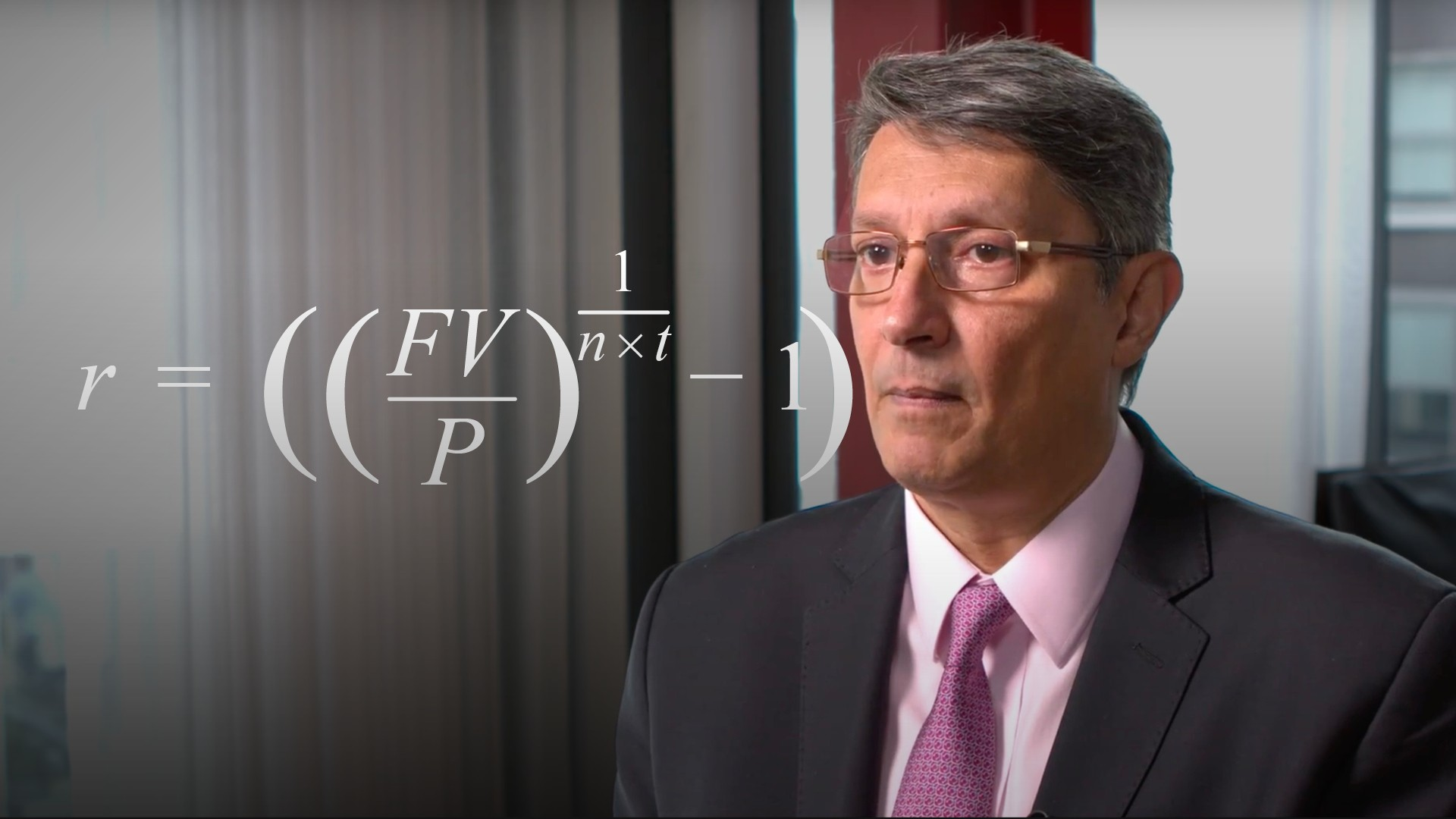Financial maths### Calculating Continuously Compounded Rates of Returns

Continuously compounded rates of return are widely used in financial markets, especially in the world of derivatives. In this video, Abdulla outlines the concept and provides some examples framed as investment returns.

Abdulla Javeri05:03

•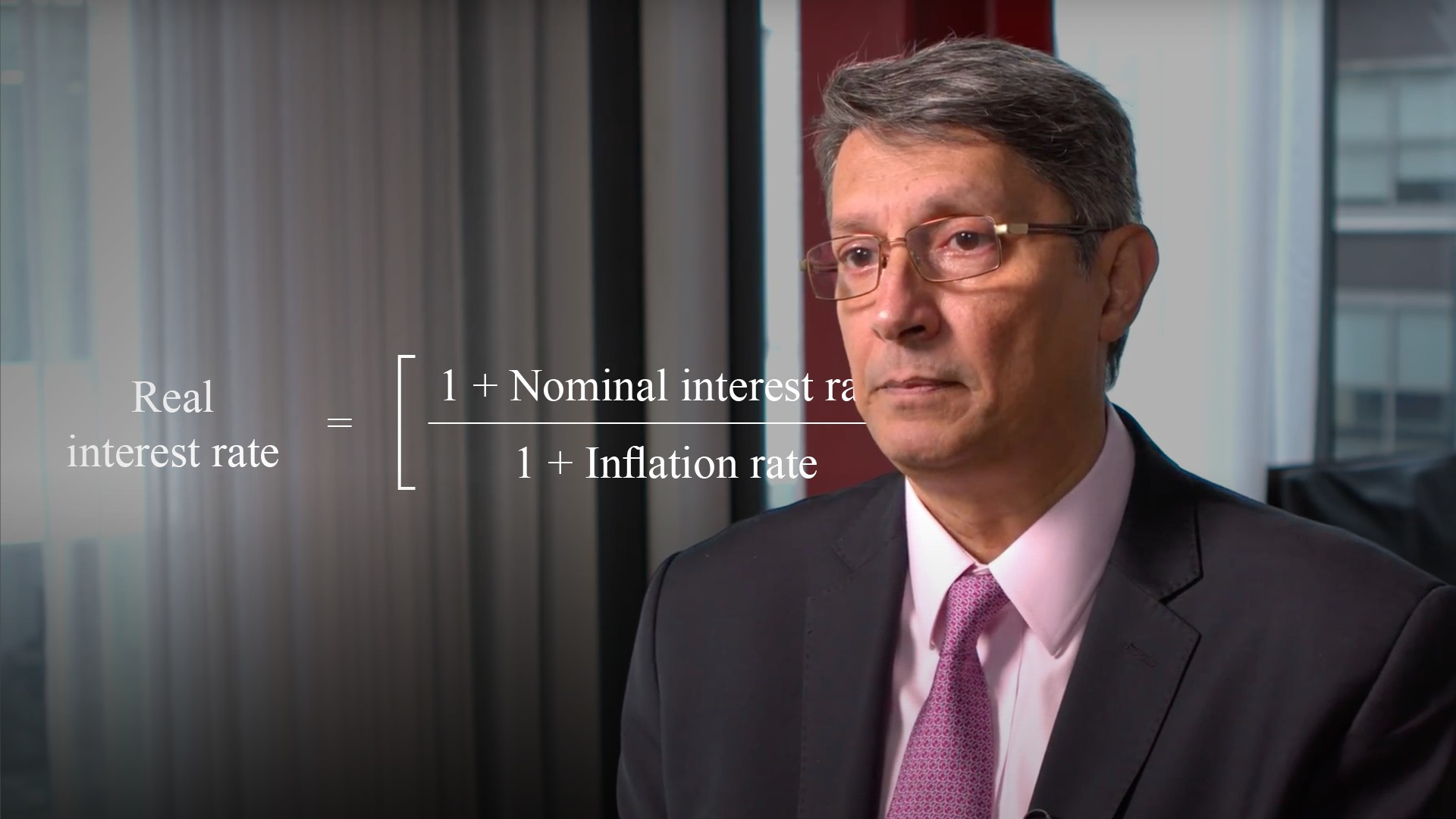Financial maths### Calculating Real Returns

Chances are when someone quotes a figure for investment returns it’s likely to be on a nominal or gross basis, without taking into account the effects of inflation. Purchasing power only increases if returns outstrip inflation over a given period. Abdulla addresses this fact and explains how to measure real returns for a single period, and over time, to help us understand inflation-adjusted returns.

Abdulla Javeri04:42

•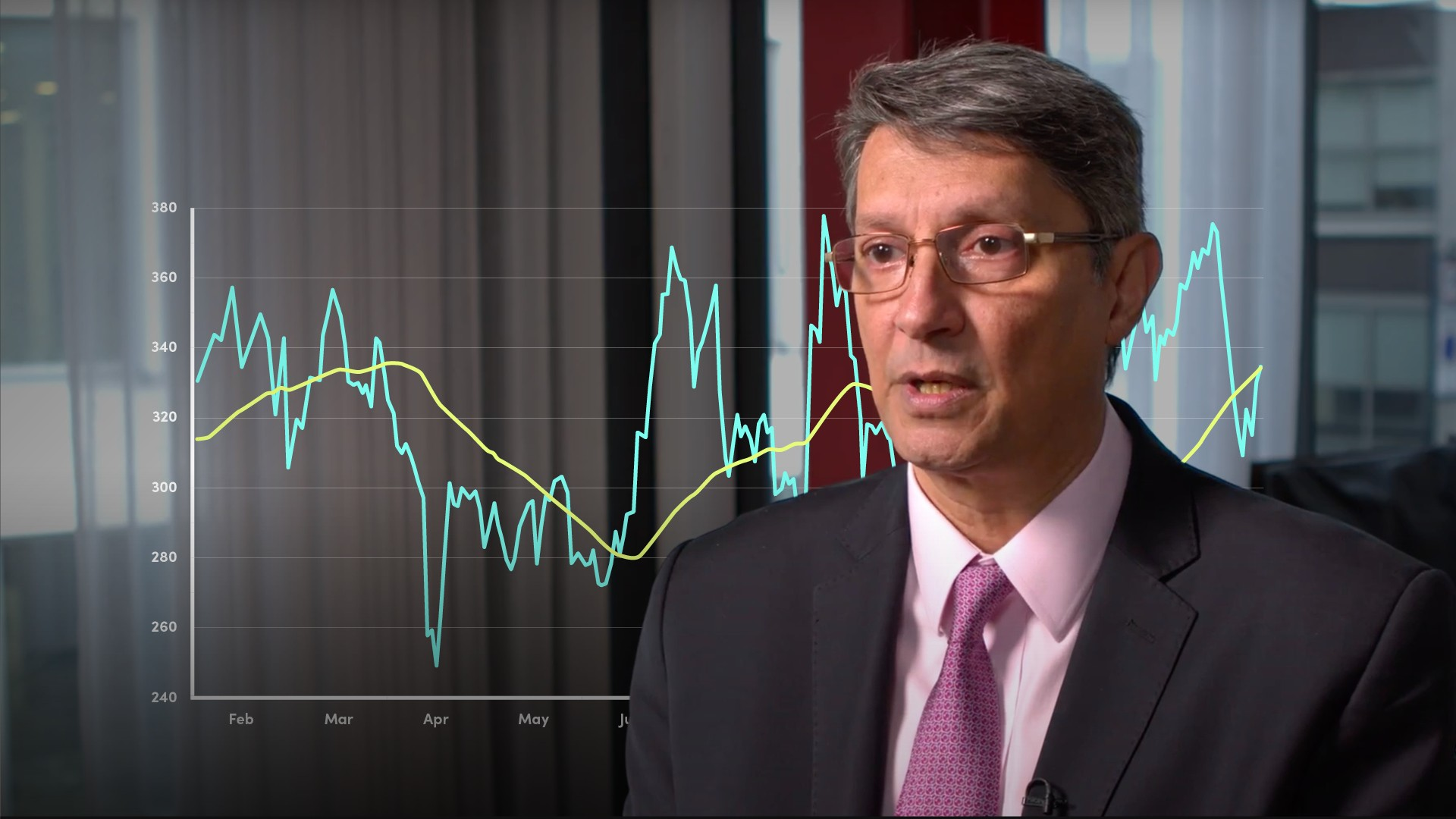Financial maths### Simple Arithmetic Moving Averages

A moving average is a rolling series of arithmetic averages which acts as a smoothing mechanism to indicate trends in historic data. In financial markets, that historic data is most likely to be prices or returns. In this video, Abdulla discusses this concept and specifically explains the simple or arithmetic moving average. He covers what it is, how it is calculated, why people use it and some of its limitations.

Abdulla Javeri04:00

•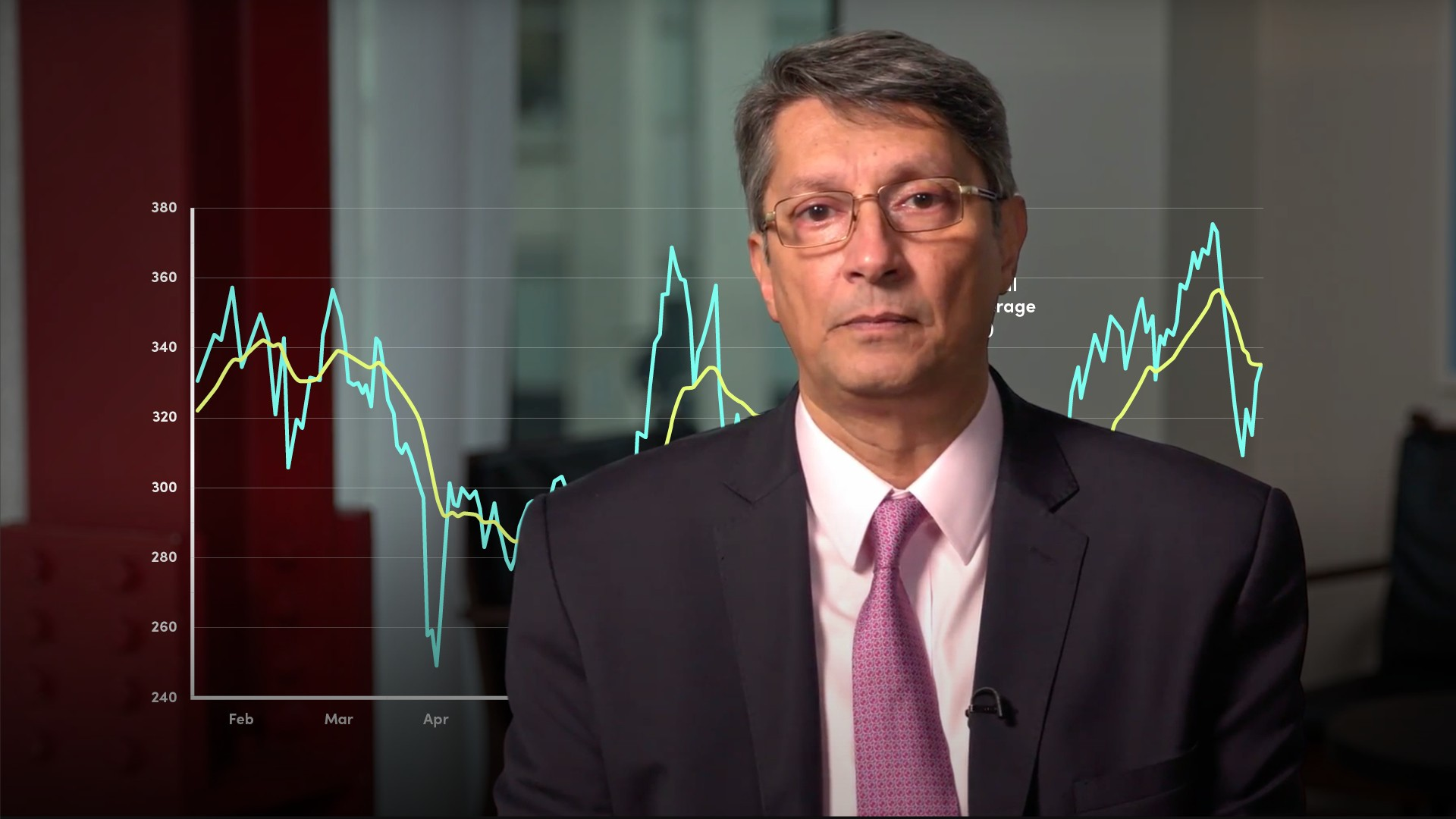Financial maths### Exponentially Weighted Moving Averages

In the previous video, Abdulla covered simple or arithmetic moving averages. Here, Abdulla explains a similar concept: the weighted and exponential moving averages. He answers the questions: what are they, how are they calculated and how are they used?

Abdulla Javeri05:11

,